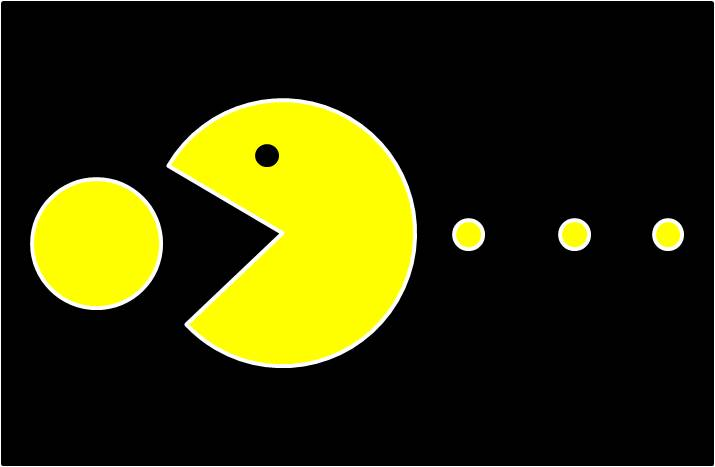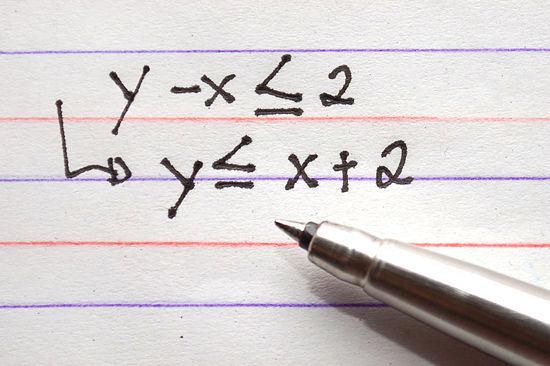Learn the 4 Things You Must Know Before Taking the GMAT!   Download it Now!

# GMAT Algebra: Inequalities and Negative NumbersOne of the algebra topics tested on the GMAT is inequalities. In may respects, inequalities are pretty simple and straightforward. As a general rule, you pretty much just treat them like normal algebraic equations when solving for the possible values of the variable(s). For example, compare the following (Equation #1 vs. Inequality #1):

Equation #1:  2x + 5 = 17

To solve, you subtract 5 from both sides and divide by 2, so x = 6.

Inequality #1:  2x + 5 < 17

To solve, you do the exact same thing — subtract 5 from both sides and divide by 2, and the inequality sign stays the same. So, x < 6.

The major difference between Inequality #1 and Equation #1 is that with the inequality, there are an infinite number of possible values for x that will satisfy the parameters set forth by the inequality. Whereas in Equation #1 there is only one value for x in the entire universe of numbers for which 2x + 5 = 17 (i.e. 6), in Inequality #1, there are multiple answers. In fact, any value of x less than 6 will work.

Pretty easy, right?

Well, it’s the GMAT after all, so you know that as the problems get more difficult, the likelihood that the test makers will lay traps for you increases. In fact, there are two major things to be on the lookout for on more difficult questions when it comes to GMAT inequalities:

### 1. Inequalities with Two VariablesInequalities that contain two variables aren’t “tricks” per se, but they do introduce an extra level of complexity that you need to make sure you know how to tackle. The good news is that it’s pretty straightforward: Inequality Tables render these types of problems harmless. Check out our article on “GMAT Math – Inequalities with Two Variables” for everything you need to know to master this important GMAT inequalities variation.

### 2. Dividing by Negative Numbers

One of the little known and often-forgotten rules of math that many GMAT students overlook is the fact that when you divide an inequality by a  negative number, the inequality sign reverses direction. In fact, let’s cement that as a rule:

### Rule: When you divide an inequality by a negative number, the inequality sign reverses direction.

Let’s look at a variation of the inequality above to see how this works:

Inequality #2: -2x + 5 < 17

The only subtle difference between Inequality #2 and Inequality #1 is that in Inequality #2, the coefficient of the variable x is negative 2. And yet, this makes all the difference in the world.

You start the same: Subtract 5 from both sides, so now -2x < 12. However, the next step is to divide both sides by negative 2 to isolate x. As the rule above suggests, this reverses the inequality sign. So, the correct answer is that x > 6, not “less than 6” as in Inequality #1 above.

This is one of those rules that you can prove to yourself by plugging a bunch of numbers in for x and looking at them on a number line and confirming to yourself that “yes,” in fact the inequality sign does reverse when you divide by a negative number. OR, you can just memorize the rule and realize that the GMAT is a means to an end, after all, and as long as knowing this rule helps you get a couple more right answers on test day, that’s all that matters. Whatever works for you.

### How This Rule Is Tested on the GMAT

This little bit of information about how negative numbers affect inequalities can be tested anywhere on the GMAT quantitative or Integrated Reasoning sections, and yet it’s most likely that you’ll encounter it on GMAT data sufficiency questions. It’s important, therefore, that you use your scratch paper and follow the methodology we lay out in “Data Sufficiency – Part 1” to make sure you don’t make a careless mistake by overlooking this subtle rule.

To see how this plays out, try your hand at this sample GMAT data sufficiency question:

If ab < ac, which is greater, b or c?

(1) a < 0
(2) c < 0

What do you think?

Well, the obvious way to proceed is to simply divide both sides of the inequality in the question stem by “a” and get that b < c. For many students, you’d stop right there and conclude that sure enough, b is less than c so you have enough information to answer the question.

The problem, of course, is that we don’t know if a is positive or negative! If a is positive, then sure enough b < c when you divide both sides of the inequality by a. However, what if a is negative? In that case, as we saw with the rule above, the inequality sign reverses because you’re now dividing the inequality by a negative number. In that case, b > c. So, we’d need further information about the nature of a to determine sufficiency.

Sure enough, the answer should now jump off the page at you:

(1) Sufficient. This is exactly what we determined in advance that we needed to know. If a < 0, then c is definitely less than b.

(2) Insufficient. The sign of c is irrelevant to answering the question.

So, the correction answer is A.

### Conclusion

The difficulty in this “trick” about the inequality sign reversing when dividing by negative numbers isn’t so much in understanding the rule itself as it is in making sure to be on the lookout for it on test day. It’s easy to be humming along on the GMAT, answering questions, and then see an inequality question and manipulate it like a normal equation without catching the subtle fact that at some point in solving the inequality you have to divide by a negative number. It’s easy to overlook. But now that you know, and now that you’ve seen this rule in action, hopefully you’ll have your antennae up and be able to beat the GMAT test makers at their own game.

In doing so, you’ll certainly be in a better position to dominate the GMAT!